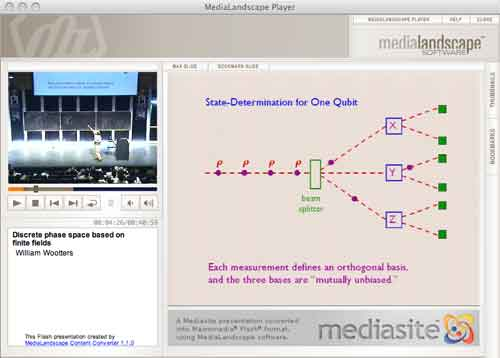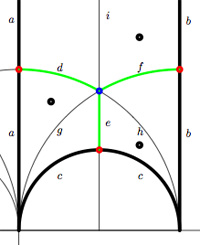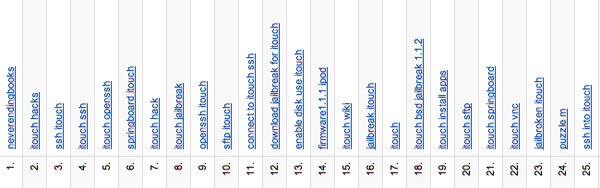# Tag: Mathieu

MUBs (for Mutually Unbiased Bases) are quite popular at the moment. Kea is running a mini-series Mutual Unbias as is Carl Brannen. Further, the Perimeter Institute has a good website for its seminars where they offer streaming video (I like their MacromediaFlash format giving video and slides/blackboard shots simultaneously, in distinct windows) including a talk on MUBs (as well as an old talk by Wootters).So what are MUBs to mathematicians? Recall that a d-state quantum system is just the vectorspace $\mathbb{C}^d$ equipped with the usual Hermitian inproduct $\vec{v}.\vec{w} = \sum \overline{v_i} w_i$. An observable $E$ is a choice of orthonormal basis ${ \vec{e_i} }$ consisting of eigenvectors of the self-adjoint matrix $E$. $E$ together with another observable $F$ (with orthonormal basis ${ \vec{f_j} }$) are said to be mutally unbiased if the norms of all inproducts $\vec{f_j}.\vec{e_i}$ are equal to $1/\sqrt{d}$. This definition extends to a collection of pairwise mutually unbiased observables. In a d-state quantum system there can be at most d+1 mutually unbiased bases and such a collection of observables is then called a MUB of the system. Using properties of finite fields one has shown that MUBs exists whenever d is a prime-power. On the other hand, existence of a MUB for d=6 still seems to be open…

The King’s Problem (( actually a misnomer, it’s more the poor physicists’ problem… )) is the following : A physicist is trapped on an island ruled by a mean
king who promises to set her free if she can give him the answer to the following puzzle. The
physicist is asked to prepare a d−state quantum system in any state of her choosing and give it
to the king, who measures one of several mutually unbiased observables on it. Following this, the physicist is allowed to make a control measurement
on the system, as well as any other systems it may have been coupled to in the preparation
phase. The king then reveals which observable he measured and the physicist is required
to predict correctly all the eigenvalues he found.

The Solution to the King’s problem in prime power dimension by P. K. Aravind, say for $d=p^k$, consists in taking a system of k object qupits (when $p=2l+1$ one qupit is a spin l particle) which she will give to the King together with k ancilla qupits that she retains in her possession. These 2k qupits are diligently entangled and prepared is a well chosen state. The final step in finding a suitable state is the solution to a pure combinatorial problem :

She must use the numbers 1 to d to form $d^2$ ordered sets of d+1 numbers each, with repetitions of numbers within a set allowed, such that any two sets have exactly one identical number in the same place in both. Here’s an example of 16 such strings for d=4 :

11432, 12341, 13214, 14123, 21324, 22413, 23142, 24231, 31243, 32134, 33421, 34312, 41111, 42222, 43333, 44444

Here again, finite fields are used in the solution. When $d=p^k$, identify the elements of $\mathbb{F}_{p^k}$ with the numbers from 1 to d in some fixed way. Then, the $d^2$ of number-strings are found as follows : let $k_0,k_1 \in \mathbb{F}_{p^k}$ and take as the first 2 numbers the ones corresponding to these field-elements. The remaning d-2 numbers in the string are those corresponding to the field element $k_m$ (with $2 \leq m \leq d$) determined from $k_0,k_1$ by the equation

$k_m = l_{m} * k_0+k_1$

where $l_i$ is the field-element corresponding to the integer i ($l_1$ corresponds to the zero element). It is easy to see that these $d^2$ strings satisfy the conditions of the combinatorial problem. Indeed, any two of its digits determine $k_0,k_1$ (and hence the whole string) as it follows from
$k_m = l_m k_0 + k_1$ and $k_r = l_r k_0 + k_1$ that $k_0 = \frac{k_m-k_r}{l_m-l_r}$.

In the special case when d=3 (that is, one spin 1 particle is given to the King), we recover the tetracode : the nine codewords

0000, 0+++, 0—, +0+-, ++-0, +-0+, -0-+, -+0-, –+0

encode the strings (with +=1,-=2,0=3)

3333, 3111, 3222, 1312, 1123, 1231, 2321, 2132, 2213

Terry Tao is reworking some of his better blogposts into a book, to be published by the AMS (here’s a preliminary version of the book “What’s New?”)

After some thought, I decided not to transcribe all of my posts from last year (there are 93 of them!), but instead to restrict attention to those articles which (a) have significant mathematical content, (b) are not announcements of material that will be published elsewhere, and (c) are not primarily based on a talk given by someone else. As it turns out, this still leaves about 33 articles from 2007, leading to a decent-sized book of a couple hundred pages in length.

If you have a blog and want to turn it into a LaTeX-book, there’s no need to transcribe or copy every single post, thanks to the WPTeX tool. Note that this is NOT a WP-plugin, but a (simple at that) php-program which turns all posts into a bookcontent.tex file. This file can then be edited further into a proper book.

Unfortunately, the present version chokes on LaTeXrender-code (which is easy enough to solve doing a global ‘find-and-replace’ of the tex-tags by dollar-signs) but worse, on Markdown-code… But then, someone fluent in php-regex will have no problems extending the libs/functions.php file (I hope…).

At the moment I’m considering turning the Mathieu-games-posts into a booklet. A possible title might be Mathieumatical Games. Rereading them (and other posts) I regret to be such an impatient blogger. Often I’m interested in something and start writing posts about it without knowing where or when I’ll land. This makes my posts a lot harder to get through than they might have been, if I would blog only after having digested the material myself… Typical recent examples are the tori-crypto-posts and the Bost-Connes algebra posts.

So, I still have a lot to learn from other bloggers I admire, such as Jennifer Ouellette who maintains the Coctail Party Physics blog. At the moment, Jennifer is resident blogger-journalist at the Kavli Institute where she is running a “Journal Club” workshop giving ideas on how to write better about science.

But the KITP is also committed to fostering scientific communication. That’s where I come in. Each Friday through April 26th, I’ll be presiding over a “Journal Club” meeting focusing on some aspect of communicating science.

Her most recent talk was entitled To Blog or Not to Blog? That is the Question and you can find the slides as well as a QuickTime movie of her talk. They even plan to set up a blog for the participants of the workshop. I will surely follow the rest of her course with keen interest!

It’s been a while, so let’s include a recap : a (transitive) permutation representation of the modular group $\Gamma = PSL_2(\mathbb{Z})$ is determined by the conjugacy class of a cofinite subgroup $\Lambda \subset \Gamma$, or equivalently, to a dessin d’enfant. We have introduced a quiver (aka an oriented graph) which comes from a triangulation of the compactification of $\mathbb{H} / \Lambda$ where $\mathbb{H}$ is the hyperbolic upper half-plane. This quiver is independent of the chosen embedding of the dessin in the Dedeking tessellation. (For more on these terms and constructions, please consult the series Modular subgroups and Dessins d’enfants).

Why are quivers useful? To start, any quiver $Q$ defines a noncommutative algebra, the path algebra $\mathbb{C} Q$, which has as a $\mathbb{C}$-basis all oriented paths in the quiver and multiplication is induced by concatenation of paths (when possible, or zero otherwise). Usually, it is quite hard to make actual computations in noncommutative algebras, but in the case of path algebras you can just see what happens.

Moreover, we can also see the finite dimensional representations of this algebra $\mathbb{C} Q$. Up to isomorphism they are all of the following form : at each vertex $v_i$ of the quiver one places a finite dimensional vectorspace $\mathbb{C}^{d_i}$ and any arrow in the quiver
$$\xymatrix{\vtx{v_i} \ar[r]^a & \vtx{v_j}}$$ determines a linear map between these vertex spaces, that is, to $a$ corresponds a matrix in $M_{d_j \times d_i}(\mathbb{C})$. These matrices determine how the paths of length one act on the representation, longer paths act via multiplcation of matrices along the oriented path.

A necklace in the quiver is a closed oriented path in the quiver up to cyclic permutation of the arrows making up the cycle. That is, we are free to choose the start (and end) point of the cycle. For example, in the one-cycle quiver

$$\xymatrix{\vtx{} \ar[rr]^a & & \vtx{} \ar[ld]^b \\ & \vtx{} \ar[lu]^c &}$$

the basic necklace can be represented as $abc$ or $bca$ or $cab$. How does a necklace act on a representation? Well, the matrix-multiplication of the matrices corresponding to the arrows gives a square matrix in each of the vertices in the cycle. Though the dimensions of this matrix may vary from vertex to vertex, what does not change (and hence is a property of the necklace rather than of the particular choice of cycle) is the trace of this matrix. That is, necklaces give complex-valued functions on representations of $\mathbb{C} Q$ and by a result of Artin and Procesi there are enough of them to distinguish isoclasses of (semi)simple representations! That is, linear combinations a necklaces (aka super-potentials) can be viewed, after taking traces, as complex-valued functions on all representations (similar to character-functions).

In physics, one views these functions as potentials and it then interested in the points (representations) where this function is extremal (minimal) : the vacua. Clearly, this does not make much sense in the complex-case but is relevant when we look at the real-case (where we look at skew-Hermitian matrices rather than all matrices). A motivating example (the Yang-Mills potential) is given in Example 2.3.2 of Victor Ginzburg’s paper Calabi-Yau algebras.

Let $\Phi$ be a super-potential (again, a linear combination of necklaces) then our commutative intuition tells us that extrema correspond to zeroes of all partial differentials $\frac{\partial \Phi}{\partial a}$ where $a$ runs over all coordinates (in our case, the arrows of the quiver). One can make sense of differentials of necklaces (and super-potentials) as follows : the partial differential with respect to an arrow $a$ occurring in a term of $\Phi$ is defined to be the path in the quiver one obtains by removing all 1-occurrences of $a$ in the necklaces (defining $\Phi$) and rearranging terms to get a maximal broken necklace (using the cyclic property of necklaces). An example, for the cyclic quiver above let us take as super-potential $abcabc$ (2 cyclic turns), then for example

$\frac{\partial \Phi}{\partial b} = cabca+cabca = 2 cabca$

(the first term corresponds to the first occurrence of $b$, the second to the second). Okay, but then the vacua-representations will be the representations of the quotient-algebra (which I like to call the vacualgebra)

$\mathcal{U}(Q,\Phi) = \frac{\mathbb{C} Q}{(\partial \Phi/\partial a, \forall a)}$

which in ‘physical relevant settings’ (whatever that means…) turn out to be Calabi-Yau algebras.

But, let us return to the case of subgroups of the modular group and their quivers. Do we have a natural super-potential in this case? Well yes, the quiver encoded a triangulation of the compactification of $\mathbb{H}/\Lambda$ and if we choose an orientation it turns out that all ‘black’ triangles (with respect to the Dedekind tessellation) have their arrow-sides defining a necklace, whereas for the ‘white’ triangles the reverse orientation makes the arrow-sides into a necklace. Hence, it makes sense to look at the cubic superpotential $\Phi$ being the sum over all triangle-sides-necklaces with a +1-coefficient for the black triangles and a -1-coefficient for the white ones. Let’s consider an index three example from a previous post$$\xymatrix{& & \rho \ar[lld]_d \ar[ld]^f \ar[rd]^e & \\ i \ar[rrd]_a & i+1 \ar[rd]^b & & \omega \ar[ld]^c \\ & & 0 \ar[uu]^h \ar@/^/[uu]^g \ar@/_/[uu]_i &}$$

In this case the super-potential coming from the triangulation is

$\Phi = -aid+agd-cge+che-bhf+bif$

and therefore we have a noncommutative algebra $\mathcal{U}(Q,\Phi)$ associated to this index 3 subgroup. Contrary to what I believed at the start of this series, the algebras one obtains in this way from dessins d’enfants are far from being Calabi-Yau (in whatever definition). For example, using a GAP-program written by Raf Bocklandt Ive checked that the growth rate of the above algebra is similar to that of $\mathbb{C}[x]$, so in this case $\mathcal{U}(Q,\Phi)$ can be viewed as a noncommutative curve (with singularities).

However, this is not the case for all such algebras. For example, the vacualgebra associated to the second index three subgroup (whose fundamental domain and quiver were depicted at the end of this post) has growth rate similar to that of $\mathbb{C} \langle x,y \rangle$…

I have an outlandish conjecture about the growth-behavior of all algebras $\mathcal{U}(Q,\Phi)$ coming from dessins d’enfants : the algebra sees what the monodromy representation of the dessin sees of the modular group (or of the third braid group).
I can make this more precise, but perhaps it is wiser to calculate one or two further examples…Kea’s post reminded me to have a look at my search terms (the things people type into search engines to get redirected here). Quite a sobering experience…

Via Google Analytics I learn that 49,51% of traffic comes from Search Engines (compared to 26,17% from Referring Sites and 24,32% from direct hits) so I should take Search Terms more seriously! Above you can find the top-25.

On 1. there is neverendingbooks. Well, some people seem to remember the blog-name, but require google to remember the URL (neverendingbooks.org)…, okay, fair enough. But from then on… all search terms are iTouch related! The first ‘other’ term is puzzle m at 24. and believe me things do not improve afterwards. Here the only non-Touch related search terms in the top 100 :

• neverendingbooks.org (40)
• “puzzle m” (42)
• moonshine mathematics (79)
• necklace algebra (80)
• “calabi-yau algebra (90)
• “dessin d enfant” (91)
• “lieven le bruyn” (95)
• Mathieu group + M(13) (97)
• 13 points 5 lines puzzle (98)
• 15 itouch sliding puzzle (99)

the last one is really touching (sic). Is there anybody out there still interested in the mathematics, or should I turn this blog into a yaib (yet another iTouch blog) ???

In a couple of days I’ll be blogging for 4 years… and I’m in the process of resurrecting about 300 posts from a database-dump made in june. For example here’s my first post ever which is rather naive. This conversion program may last for a couple of weeks and I apologize for all unwanted pingbacks it will produce.

I’ll try to convert chunks of related posts in one go, so that I can at least give them correct self-references. Today’s work consisted in rewriting the posts of my virtual course, in march of this year, on dessins d’enfants and its connection to noncommutative geometry (a precursor of what Ive been blogging about recently). These posts were available through the PDF-archive but are from now on open to the internal search-function. Here are the internal links and a short description of their contents

Besides, I’ve added a few scattered old posts, many more to follow…